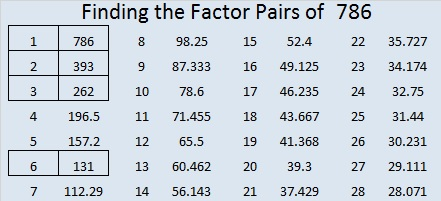# 786 and Level 5

786 is even so it is divisible by 2. Also since 786 is made from 3 consecutive numbers, we can tell automatically that it is divisible by 3. Those two facts together mean 786 is also divisible by 6.

• 786 is a composite number.
• Prime factorization: 786 = 2 x 3 x 131
• The exponents in the prime factorization are 1, 1, and 1. Adding one to each and multiplying we get (1 + 1)(1 + 1)(1 + 1) = 2 x 2 x 2 = 8. Therefore 786 has exactly 8 factors.
• Factors of 786: 1, 2, 3, 6, 131, 262, 393, 786
• Factor pairs: 786 = 1 x 786, 2 x 393, 3 x 262, or 6 x 131
• 786 has no square factors that allow its square root to be simplified. √786 ≈ 28.03569.Today’s Find the Factors puzzle:Print the puzzles or type the solution on this excel file: 12-factors-782-787

———————————

Here’s a little more about the number 786:

786 is 123 in BASE 27 because 1(27²) + 2(27) + 3(1) = 786.

786 is the sum of two consecutive primes: 389 + 397 = 786

———————————This site uses Akismet to reduce spam. Learn how your comment data is processed.